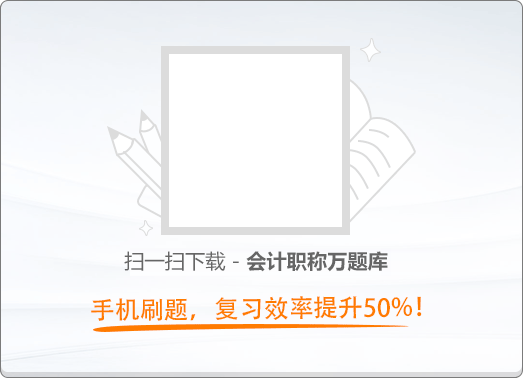90:002012年经济法基础真题
2012年初级会计职称《经济法基础》考试真题
1
(单项选择题)

• A.

机动车辆

• B.

金银首饰

• C.

古玩字画

• D.

维持生活必需的住房

• A
• B
• C
• D

2
(单项选择题)

• A.

处以2 000元以下的罚款

• B.

没收经营所得

• C.

提请司法机关查封财产

• D.

提请工商行政管理机关吊销营业执照

• A
• B
• C
• D

3
(单项选择题)

• A.

党、团、工会经费专用存款账户

• B.

个人银行结算账户

• C.

预算单位零余额账户

• D.

单位银行卡账户

• A
• B
• C
• D

4
(单项选择题)

• A.

1 000

• B.

1 500

• C.

2 000

• D.

2 500

• A
• B
• C
• D

5
(单项选择题)

• A.

粘单上第一手背书的背书人

• B.

票据上最后一手背书的背书人

• C.

票据上第一手背书的背书人

• D.

粘单上第一手背书的被背书人

• A
• B
• C
• D

6
(单项选择题)

• A.

假冒出票人在票据上签章

• B.

涂改票据号码

• C.

对票据金额进行挖补篡改

• D.

修改票据密押

• A
• B
• C
• D

7
(单项选择题)

• A.

宪法

• B.

行政法规

• C.

法律

• D.

行政规章

• A
• B
• C
• D

8
(单项选择题)

• A.

2年

• B.

5年

• C.

20年

• D.

30年

• A
• B
• C
• D

9
(单项选择题)

• A.

15天以下

• B.

1个月以上6个月以下

• C.

3个月以上2年以下

• D.

6个月以上15年以下

• A
• B
• C
• D

10
(单项选择题)

• A.

0

• B.

2 000

• C.

22 000

• D.

24 000

• A
• B
• C
• D

11
(单项选择题)

• A.

2012年6月15日

• B.

2012年7月2日

• C.

2012年7月15日

• D.

2012年8月2日

• A
• B
• C
• D

12
(单项选择题)

• A.

不低于职工本人小时工资标准的100%

• B.

不低于职工本人小时工资标准的150%

• C.

不低于职工本人小时工资标准的200%

• D.

不低于职工本人小时工资标准的300%

• A
• B
• C
• D

13
(单项选择题)

• A.

20 000

• B.

24 000

• C.

60 000

• D.

70 000

• A
• B
• C
• D

14
(单项选择题)

• A.

体育业

• B.

财产保险业务

• C.

旅游业

• D.

修理修配业务

• A
• B
• C
• D

15
(单项选择题)

• A.

154×5%＝7.7万元

• B.

（154＋20）×5%＝8.7万元

• C.

（154＋20＋25）×5%＝9.95万元

• D.

（154＋20＋25－120）×5%＝3.95万元

• A
• B
• C
• D

16
(单项选择题)

• A.

退休人员再任职取得的收入

• B.

从非任职公司取得的董事费收入

• C.

从任职公司取得的监事费收入

• D.

从任职公司关联企业取得的监事费收入

• A
• B
• C
• D

17
(单项选择题)

• A.

1 840

• B.

1 600

• C.

2 000

• D.

4 800

• A
• B
• C
• D

18
(单项选择题)

• A.

加班工资

• B.

独生子女补贴

• C.

差旅费津贴

• D.

国债利息收入

• A
• B
• C
• D

19
(单项选择题)

• A.

房屋出租的，承租人为纳税人

• B.

房屋产权所有人不在房产所在地的，房产代管人为纳税人

• C.

房屋产权属于国家的，其经营管理单位为纳税人

• D.

房屋产权未确定的，房产代管人为纳税人

• A
• B
• C
• D

20
(单项选择题)

• A.

12 000×（1－30%）×1.2%＝100.8万元

• B.

12 000×（1－30%）×1.2%＋80×12%＝110.4万元

• C.

（12 000＋400）×（1－30%）×1.2%＋80×12%＝113.76万元

• D.

（12 000＋400＋600）×（1－30%）×1.2%＝109.2万元

• A
• B
• C
• D

21
(单项选择题)

• A.

15

• B.

45

• C.

60

• D.

75

• A
• B
• C
• D

22
(单项选择题)

• A.

载客汽车

• B.

银行运钞车

• C.

机关公务车

• D.

养殖渔船

• A
• B
• C
• D

23
(单项选择题)

• A.

甲和乙为纳税人

• B.

甲和丙为纳税人

• C.

乙和丁为纳税人

• D.

甲和丁为纳税人

• A
• B
• C
• D

24
(单项选择题)

• A.

加收税款滞纳金

• B.

没收财物和违法所得

• C.

罚款

• D.

停止发售发票

• A
• B
• C
• D